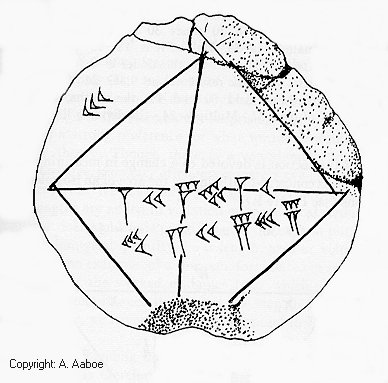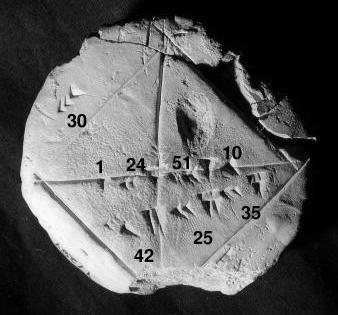#Drama! Intrigue! Geekiness!

## September 19, 2007

### By the rivers of…

What is this? And what is its claim to fame?this may help a bit moreUnearthed by: Abhishek , ramsaravana , nihit , Gammafunction , Vinod , uma , Sharath , Swetha , Arjun June , Tathagata Chatterjee , shivani , yaksha , srichand.

This is the famous root(2) tablet, pretty much the first known reference to an irrational number. See Abhishek or Yaksha’s comment for more details :)

### 16 Responses to “By the rivers of…”

1.  First example of cuniform writing from mesopotemia. perhaps first example of symbolic writing ?

2. Piyush
 The first lunar table? (found in Babylon)

3. AbhishekThe famous ‘root(2)’ tablet from the Yale Babylonian Collection. . This tablet consists of a drawing of a square with its diagonals also included. Next to one side of the square is the Babylonian representation of our number 30. Near the intersection of the diagonals are the numbers we would represent as 1.41421297 and below that 42.4263891. No explanation is given. However, based on the geometry and how close the number 1.41421297 is to 2 (1.41421356 to the same number of places), it is clear that the tablet shows how to calculate the diagonal of the square by giving a value for 2 and 30 2 , which would be the length of a diagonal of a 30 by 30 square. In addition to showing that the Babylonians knew of the Pythagorean theorem, over 1000 years before Pythagoras lived, it also shows an extremely accurate approximation of 2 .

4.  Square root of 2… sq root of 2 is 1 + 24/60 + 51/60^2 and so on…………..

5.  Square root of 2…..

6. nihitBabylonian clay tablet YBC 7289… The Babylonian clay tablet YBC 7289 (c. 1800–1600 BCE) gives an approximation of suqare root of 2 in four sexagesimal figures, which is about six decimal figures. I do not understand too much more…

7. GammafunctionBabylonian clay tablet giving an approximation of root 2 in sexagesimal format.

8. Vinod
 Babylonian clay tablet YBC 7289 with annotations. The diagonal displays an approximation of the square root of 2 in four sexagesimal figures, which is about six decimal figures. The Babylonian system of mathematics was sexagesimal (base-60) numeral system. From this we derive the modern day usage of 60 seconds in a minute, 60 minutes in an hour, and 360 (60×6) degrees in a circle. Our knowledge of Babylonian mathematics is derived from some 400 clay tablets unearthed since the 1850s. Written in Cuneiform script, tablets were inscribed whilst the clay was moist, and baked hard in an oven or by the heat of the sun. The majority of recovered clay tablets date from 1800 to 1600 BC, and cover topics which include fractions, algebra, quadratic and cubic equations, the Pythagorean theorem, and the calculation of Pythagorean triples and possibly trigonometric functions.

9.  by the rivers of babylon…..the mention of asger aaboe gave it away both the pics are the famous ‘root(2)’ tablet from the Yale Babylonian Collection. This Babylonian cuneiform tablet YBC7289, gives the square root of 2 as 1, 24, 51, 10 = 1 + 24/60 + 51/60×60 + 10/60x60x60. for further study refer: http://it.stlawu.edu/~dmelvill.....C7289.html

10. Sharath
 It is the ‘sexagesimal Babylonian cuneiform system’ depicted in the face of a clay tablet from the Yale Babylonian Collection (7289). Across the diagonal is written 1 24 51 10 = 1 + 24/60 + 51/3600 + 10/216000, which is 1.41421296 to 9 significant decimal figures. The true 9-figure approximation to the square root of 2 is 1.41421356! The 30 at upper left presumably represents a somewhat arbitrary dimension assigned to the side of the square. The length of the diagonal, on this assumption, is 30 times the square root of 2, which is 45 25 35 in base 60. Thus,the Babylonians knew that the ratio of diagonal to side was exactly the square root of 2.

11. Swetha
 This is the root(2) tablet from the Yale Babylonian Collection found near the Euphrates River dating from around 2000 B.C.

12. Arjun June
 Babylonian clay tablet, with annotations in Cuneiform script…The diagonal displays an approximation of the square root of 2 1 + 24/60 + 51/(60*60) + 10/(60*60*60) = 1.41421….

13. Tathagata ChatterjeeThis is the Babylonian Clay Tablet ( YBC 7289). Its claim to fame is the fact that it is the world’s first representation of sexagesimal approximation of the number “square root of 2”- the length of the diagonal of a unit square.This has wide reaching consequences, in the field of Numerical analysis, used nowadays for example : Stock markets regards, Tathagata Chatterjee

14. shivani
 its the yale tablet YBC-7289…The famous ‘root(2)’ tablet from the Yale Babylonian Collection.significance:the Babylonians found this remarkably good approximation to √2.it also proves dat babylonians knew about pythagores theorem.

15. yakshaThe famous ‘root(2)’ tablet from the Yale Babylonian Collection. [wiki rip] Babylonian clay tablet YBC 7289 with annotations. The diagonal displays an approximation of the square root of 2 in four sexagesimal figures, which is about six decimal figures. 1 + 24/60 + 51/602 + 10/603 = 1.41421296… (Image by Bill Casselman) [/wiki rip]

16.  Rivers of Babylon! The picture is that of a clay tablet recovered from excavations of the sites of ancient babylonian civilizations. The tablet describes base-60 mathematics, the base from which current time is derived. The numbers “1 24 51 10” indicate the square root of 2 in base-60 The numbers 42, 25 and 35 are the lengths of the sides of a square as marked. After appropriate conversions, the length of the diagonal is ~ 1.414, which is root 2. And this was some years before pythagoras! The numbers are more appropriately called Babylonian Pythagorean Triplets For those interested in reading Babylonian mathematical tablets: http://www.math.ubc.ca/~cass/c......html#read

« Previous « No wires attached.. « | » psst.. your (censored) are showing! » Next »